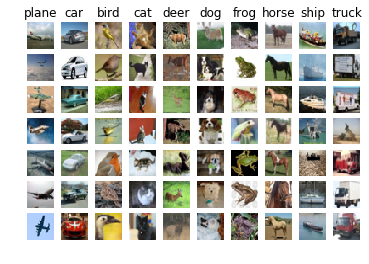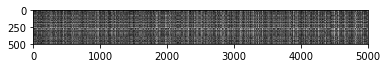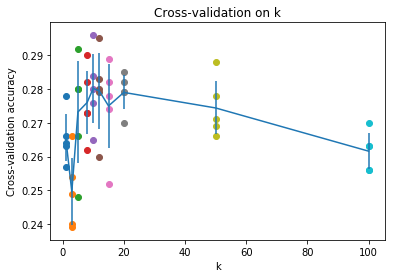# cs231n 2019. A1, part 1. k-Nearest Neighbor (kNN) exercise#### Solution by Yury Kashnitsky (@yorko)

Complete and hand in this completed worksheet (including its outputs and any supporting code outside of the worksheet) with your assignment submission. For more details see the assignments page on the course website.

The kNN classifier consists of two stages:

• During training, the classifier takes the training data and simply remembers it
• During testing, kNN classifies every test image by comparing to all training images and transfering the labels of the k most similar training examples
• The value of k is cross-validated

In this exercise you will implement these steps and understand the basic Image Classification pipeline, cross-validation, and gain proficiency in writing efficient, vectorized code.

In :
# Run some setup code for this notebook.

import random
import numpy as np
from tqdm import tqdm_notebook
import matplotlib.pyplot as plt

# This is a bit of magic to make matplotlib figures appear inline in the notebook
# rather than in a new window.
%matplotlib inline
plt.rcParams['figure.figsize'] = (10.0, 8.0) # set default size of plots
plt.rcParams['image.interpolation'] = 'nearest'
plt.rcParams['image.cmap'] = 'gray'

# Some more magic so that the notebook will reload external python modules;

In :
# Load the raw CIFAR-10 data.
cifar10_dir = '../cs231n/datasets/cifar-10-batches-py'

# Cleaning up variables to prevent loading data multiple times (which may cause memory issue)
try:
del X_train, y_train
del X_test, y_test
except:
pass

X_train, y_train, X_test, y_test = load_CIFAR10(cifar10_dir)

# As a sanity check, we print out the size of the training and test data.
print('Training data shape: ', X_train.shape)
print('Training labels shape: ', y_train.shape)
print('Test data shape: ', X_test.shape)
print('Test labels shape: ', y_test.shape)

Training data shape:  (50000, 32, 32, 3)
Training labels shape:  (50000,)
Test data shape:  (10000, 32, 32, 3)
Test labels shape:  (10000,)

In :
# Visualize some examples from the dataset.
# We show a few examples of training images from each class.
classes = ['plane', 'car', 'bird', 'cat', 'deer', 'dog', 'frog', 'horse', 'ship', 'truck']
num_classes = len(classes)
samples_per_class = 7
for y, cls in enumerate(classes):
idxs = np.flatnonzero(y_train == y)
idxs = np.random.choice(idxs, samples_per_class, replace=False)
for i, idx in enumerate(idxs):
plt_idx = i * num_classes + y + 1
plt.subplot(samples_per_class, num_classes, plt_idx)
plt.imshow(X_train[idx].astype('uint8'))
plt.axis('off')
if i == 0:
plt.title(cls)
plt.show()In :
# Subsample the data for more efficient code execution in this exercise
num_training = 5000

num_test = 500

# Reshape the image data into rows
X_train = np.reshape(X_train, (X_train.shape, -1))
X_test = np.reshape(X_test, (X_test.shape, -1))
print(X_train.shape, X_test.shape)

(5000, 3072) (500, 3072)

In :
from cs231n.classifiers import KNearestNeighbor

# Create a kNN classifier instance.
# Remember that training a kNN classifier is a noop:
# the Classifier simply remembers the data and does no further processing
classifier = KNearestNeighbor()
classifier.train(X_train, y_train)


We would now like to classify the test data with the kNN classifier. Recall that we can break down this process into two steps:

1. First we must compute the distances between all test examples and all train examples.
2. Given these distances, for each test example we find the k nearest examples and have them vote for the label

Lets begin with computing the distance matrix between all training and test examples. For example, if there are Ntr training examples and Nte test examples, this stage should result in a Nte x Ntr matrix where each element (i,j) is the distance between the i-th test and j-th train example.

Note: For the three distance computations that we require you to implement in this notebook, you may not use the np.linalg.norm() function that numpy provides.

First, open cs231n/classifiers/k_nearest_neighbor.py and implement the function compute_distances_two_loops that uses a (very inefficient) double loop over all pairs of (test, train) examples and computes the distance matrix one element at a time.

In :
# Open cs231n/classifiers/k_nearest_neighbor.py and implement
# compute_distances_two_loops.

dists = classifier.compute_distances_two_loops(X_test)
print(dists.shape)

(500, 5000)

In :
# We can visualize the distance matrix: each row is a single test example and
# its distances to training examples
plt.imshow(dists, interpolation='none')
plt.show()Inline Question 1

Notice the structured patterns in the distance matrix, where some rows or columns are visible brighter. (Note that with the default color scheme black indicates low distances while white indicates high distances.)

• What in the data is the cause behind the distinctly bright rows?
• What causes the columns?

$\color{blue}{\textit Your Answer:}$ Bright rows and columns indicate outliers, i.e. images that a far away in terms of the L2-norm from all other images.

In :
# Now implement the function predict_labels and run the code below:
# We use k = 1 (which is Nearest Neighbor).
y_test_pred = classifier.predict_labels(dists, k=1)

# Compute and print the fraction of correctly predicted examples
num_correct = np.sum(y_test_pred == y_test)
accuracy = float(num_correct) / num_test
print('Got %d / %d correct => accuracy: %f' % (num_correct, num_test, accuracy))

Got 137 / 500 correct => accuracy: 0.274000


You should expect to see approximately 27% accuracy. Now lets try out a larger k, say k = 5:

In :
y_test_pred = classifier.predict_labels(dists, k=5)
num_correct = np.sum(y_test_pred == y_test)
accuracy = float(num_correct) / num_test
print('Got %d / %d correct => accuracy: %f' % (num_correct, num_test, accuracy))

Got 139 / 500 correct => accuracy: 0.278000


You should expect to see a slightly better performance than with k = 1.

Inline Question 2

We can also use other distance metrics such as L1 distance. For pixel values $p_{ij}^{(k)}$ at location $(i,j)$ of some image $I_k$,

the mean $\mu$ across all pixels over all images is $$\mu=\frac{1}{nhw}\sum_{k=1}^n\sum_{i=1}^{h}\sum_{j=1}^{w}p_{ij}^{(k)}$$ And the pixel-wise mean $\mu_{ij}$ across all images is $$\mu_{ij}=\frac{1}{n}\sum_{k=1}^np_{ij}^{(k)}.$$ The general standard deviation $\sigma$ and pixel-wise standard deviation $\sigma_{ij}$ is defined similarly.

Which of the following preprocessing steps will not change the performance of a Nearest Neighbor classifier that uses L1 distance? Select all that apply.

1. Subtracting the mean $\mu$ ($\tilde{p}_{ij}^{(k)}=p_{ij}^{(k)}-\mu$.)
2. Subtracting the per pixel mean $\mu_{ij}$ ($\tilde{p}_{ij}^{(k)}=p_{ij}^{(k)}-\mu_{ij}$.)
3. Subtracting the mean $\mu$ and dividing by the standard deviation $\sigma$.
4. Subtracting the pixel-wise mean $\mu_{ij}$ and dividing by the pixel-wise standard deviation $\sigma_{ij}$.
5. Rotating the coordinate axes of the data.

$\color{blue}{\textit Your Answer:} 1, 2, 3, 5$

$\color{blue}{\textit Your Explanation:}$

$L_1$-distance between images $I_l$ and $I_m$: $$L_1(I_l, I_m) = \sum_{i=1}^{h}\sum_{j=1}^{w}|p_{ij}^{(l)} - p_{ij}^{(m)}|$$

1. $$\tilde{L}_1(I_l, I_m) = \sum_{i=1}^{h}\sum_{j=1}^{w}|\tilde{p}_{ij}^{(l)} - \tilde{p}_{ij}^{(m)}| = \sum_{i=1}^{h}\sum_{j=1}^{w}|(p_{ij}^{(l)} - \mu) - (p_{ij}^{(m)} - \mu)| = L_1(I_l, I_m),$$ no difference

2. $$\tilde{L}_1(I_l, I_m) = \sum_{i=1}^{h}\sum_{j=1}^{w}|\tilde{p}_{ij}^{(l)} - \tilde{p}_{ij}^{(m)}| = \sum_{i=1}^{h}\sum_{j=1}^{w}|(p_{ij}^{(l)} - \mu_{ij}) - (p_{ij}^{(m)} - \mu_{ij})| = L_1(I_l, I_m),$$ no difference

3. $$\tilde{L}_1(I_l, I_m) = \sum_{i=1}^{h}\sum_{j=1}^{w}|\tilde{p}_{ij}^{(l)} - \tilde{p}_{ij}^{(m)}| = \sum_{i=1}^{h}\sum_{j=1}^{w}|\frac{p_{ij}^{(l)} - \mu}{\sigma} - \frac{p_{ij}^{(m)} - \mu}{\sigma}| = \frac{1}{\sigma}L_1(I_l, I_m)$$ All distances between images are shrinked by a factor of $\sigma$, but it will not affect classification, because all distances are changed in the same way

4. $$\tilde{L}_1(I_l, I_m) = \sum_{i=1}^{h}\sum_{j=1}^{w}|\tilde{p}_{ij}^{(l)} - \tilde{p}_{ij}^{(m)}| = \sum_{i=1}^{h}\sum_{j=1}^{w}|\frac{p_{ij}^{(l)} - \mu_{ij}}{\sigma_{ij}} - \frac{p_{ij}^{(m)} - \mu_{ij}}{\sigma_{ij}}| = \sum_{i=1}^{h}\sum_{j=1}^{w}|\frac{p_{ij}^{(l)} - p_{ij}^{(m)}}{\sigma_{ij}}| \neq L_1(I_l, I_m),$$ In this case results classification results will change

5. Rotation will not affect distances

In :
# Now lets speed up distance matrix computation by using partial vectorization
# with one loop. Implement the function compute_distances_one_loop and run the
# code below:
dists_one = classifier.compute_distances_one_loop(X_test)

# To ensure that our vectorized implementation is correct, we make sure that it
# agrees with the naive implementation. There are many ways to decide whether
# two matrices are similar; one of the simplest is the Frobenius norm. In case
# you haven't seen it before, the Frobenius norm of two matrices is the square
# root of the squared sum of differences of all elements; in other words, reshape
# the matrices into vectors and compute the Euclidean distance between them.
difference = np.linalg.norm(dists - dists_one, ord='fro')
print('One loop difference was: %f' % (difference, ))
if difference < 0.001:
print('Good! The distance matrices are the same')
else:
print('Uh-oh! The distance matrices are different')

One loop difference was: 0.000000
Good! The distance matrices are the same

In :
# Now implement the fully vectorized version inside compute_distances_no_loops
# and run the code
dists_two = classifier.compute_distances_no_loops(X_test)

# check that the distance matrix agrees with the one we computed before:
difference = np.linalg.norm(dists - dists_two, ord='fro')
print('No loop difference was: %f' % (difference, ))
if difference < 0.001:
print('Good! The distance matrices are the same')
else:
print('Uh-oh! The distance matrices are different')

No loop difference was: 0.000000
Good! The distance matrices are the same

In :
# Let's compare how fast the implementations are
def time_function(f, *args):
"""
Call a function f with args and return the time (in seconds) that it took to execute.
"""
import time
tic = time.time()
f(*args)
toc = time.time()

two_loop_time = time_function(classifier.compute_distances_two_loops, X_test)
print('Two loop version took %f seconds' % two_loop_time)

one_loop_time = time_function(classifier.compute_distances_one_loop, X_test)
print('One loop version took %f seconds' % one_loop_time)

no_loop_time = time_function(classifier.compute_distances_no_loops, X_test)
print('No loop version took %f seconds' % no_loop_time)

# You should see significantly faster performance with the fully vectorized implementation!

# NOTE: depending on what machine you're using,
# you might not see a speedup when you go from two loops to one loop,
# and might even see a slow-down.

Two loop version took 30.631023 seconds
One loop version took 59.881897 seconds
No loop version took 0.405085 seconds


### Cross-validation¶

We have implemented the k-Nearest Neighbor classifier but we set the value k = 5 arbitrarily. We will now determine the best value of this hyperparameter with cross-validation.

In :
num_folds = 5
k_choices = [1, 3, 5, 8, 10, 12, 15, 20, 50, 100]

X_train_folds = []
y_train_folds = []
################################################################################
# TODO:                                                                        #
# Split up the training data into folds. After splitting, X_train_folds and    #
# y_train_folds should each be lists of length num_folds, where                #
# y_train_folds[i] is the label vector for the points in X_train_folds[i].     #
# Hint: Look up the numpy array_split function.                                #
################################################################################
# *****START OF YOUR CODE (DO NOT DELETE/MODIFY THIS LINE)*****

split_idx = np.array_split(range(X_train.shape), num_folds)

X_train_folds = np.array([X_train[train_idx] for train_idx in split_idx])
y_train_folds = np.array([y_train[train_idx].reshape(-1,1)
for train_idx in split_idx])

# *****END OF YOUR CODE (DO NOT DELETE/MODIFY THIS LINE)*****

# A dictionary holding the accuracies for different values of k that we find
# when running cross-validation. After running cross-validation,
# k_to_accuracies[k] should be a list of length num_folds giving the different
# accuracy values that we found when using that value of k.
k_to_accuracies = {}

################################################################################
# TODO:                                                                        #
# Perform k-fold cross validation to find the best value of k. For each        #
# possible value of k, run the k-nearest-neighbor algorithm num_folds times,   #
# where in each case you use all but one of the folds as training data and the #
# last fold as a validation set. Store the accuracies for all fold and all     #
# values of k in the k_to_accuracies dictionary.                               #
################################################################################
# *****START OF YOUR CODE (DO NOT DELETE/MODIFY THIS LINE)*****

knn = KNearestNeighbor()

for k in tqdm_notebook(k_choices):
accuracies = []
for i in range(num_folds):
all_other_fold_idx = list(set(range(num_folds)).difference([i]))
X_train_cv = np.vstack(X_train_folds[all_other_fold_idx])
y_train_cv = np.vstack(y_train_folds[all_other_fold_idx]).flatten()
X_valid_cv = X_train_folds[i]
y_valid_cv = y_train_folds[i].flatten()

knn.train(X_train_cv, y_train_cv)

knn_valid_pred = knn.predict(X_valid_cv, k=k, num_loops=0)

num_correct = np.sum(knn_valid_pred == y_valid_cv)
accuracy = float(num_correct) / y_valid_cv.shape

accuracies.append(accuracy)

k_to_accuracies[k] = accuracies

# *****END OF YOUR CODE (DO NOT DELETE/MODIFY THIS LINE)*****

# Print out the computed accuracies
for k in sorted(k_to_accuracies):
for accuracy in k_to_accuracies[k]:
print('k = %d, accuracy = %f' % (k, accuracy))

k = 1, accuracy = 0.263000
k = 1, accuracy = 0.257000
k = 1, accuracy = 0.264000
k = 1, accuracy = 0.278000
k = 1, accuracy = 0.266000
k = 3, accuracy = 0.239000
k = 3, accuracy = 0.249000
k = 3, accuracy = 0.240000
k = 3, accuracy = 0.266000
k = 3, accuracy = 0.254000
k = 5, accuracy = 0.248000
k = 5, accuracy = 0.266000
k = 5, accuracy = 0.280000
k = 5, accuracy = 0.292000
k = 5, accuracy = 0.280000
k = 8, accuracy = 0.262000
k = 8, accuracy = 0.282000
k = 8, accuracy = 0.273000
k = 8, accuracy = 0.290000
k = 8, accuracy = 0.273000
k = 10, accuracy = 0.265000
k = 10, accuracy = 0.296000
k = 10, accuracy = 0.276000
k = 10, accuracy = 0.284000
k = 10, accuracy = 0.280000
k = 12, accuracy = 0.260000
k = 12, accuracy = 0.295000
k = 12, accuracy = 0.279000
k = 12, accuracy = 0.283000
k = 12, accuracy = 0.280000
k = 15, accuracy = 0.252000
k = 15, accuracy = 0.289000
k = 15, accuracy = 0.278000
k = 15, accuracy = 0.282000
k = 15, accuracy = 0.274000
k = 20, accuracy = 0.270000
k = 20, accuracy = 0.279000
k = 20, accuracy = 0.279000
k = 20, accuracy = 0.282000
k = 20, accuracy = 0.285000
k = 50, accuracy = 0.271000
k = 50, accuracy = 0.288000
k = 50, accuracy = 0.278000
k = 50, accuracy = 0.269000
k = 50, accuracy = 0.266000
k = 100, accuracy = 0.256000
k = 100, accuracy = 0.270000
k = 100, accuracy = 0.263000
k = 100, accuracy = 0.256000
k = 100, accuracy = 0.263000

In :
# plot the raw observations
for k in k_choices:
accuracies = k_to_accuracies[k]
plt.scatter([k] * len(accuracies), accuracies)

# plot the trend line with error bars that correspond to standard deviation
accuracies_mean = np.array([np.mean(v) for k,v in sorted(k_to_accuracies.items())])
accuracies_std = np.array([np.std(v) for k,v in sorted(k_to_accuracies.items())])
plt.errorbar(k_choices, accuracies_mean, yerr=accuracies_std)
plt.title('Cross-validation on k')
plt.xlabel('k')
plt.ylabel('Cross-validation accuracy')
plt.show()In :
# Based on the cross-validation results above, choose the best value for k,
# retrain the classifier using all the training data, and test it on the test
# data. You should be able to get above 28% accuracy on the test data.
best_k = 1

classifier = KNearestNeighbor()
classifier.train(X_train, y_train)
y_test_pred = classifier.predict(X_test, k=best_k)

# Compute and display the accuracy
num_correct = np.sum(y_test_pred == y_test)
accuracy = float(num_correct) / num_test
print('Got %d / %d correct => accuracy: %f' % (num_correct, num_test, accuracy))

Got 137 / 500 correct => accuracy: 0.274000


Inline Question 3

Which of the following statements about $k$-Nearest Neighbor ($k$-NN) are true in a classification setting, and for all $k$? Select all that apply.

1. The decision boundary of the k-NN classifier is linear.
2. The training error of a 1-NN will always be lower than that of 5-NN.
3. The test error of a 1-NN will always be lower than that of a 5-NN.
4. The time needed to classify a test example with the k-NN classifier grows with the size of the training set.
5. None of the above.

$\color{blue}{\textit Your Answer:}$ 2,4

$\color{blue}{\textit Your Explanation:}$

• 1 is incorrect - the boundary is highly non-linear
• 2 is correct - for 1-NN, training error is always 0
• 3 is incorrect - typically, 5-NN will work better
• 4 is correct - test time complexity is proportional to the training set length